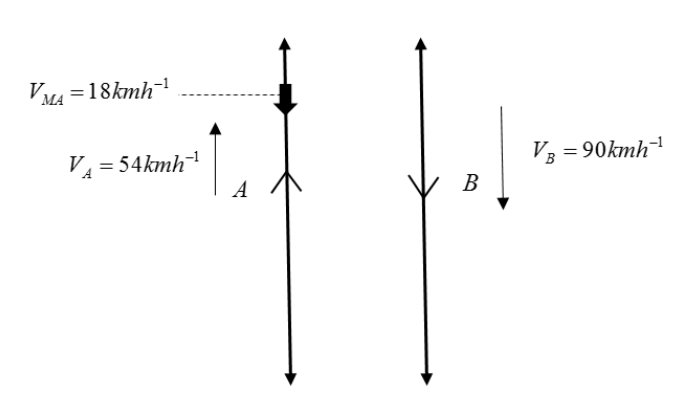QuestionAnswers

# Two parallel rail tracks run north-south. Train $A$ moves north with a speed of $54km{{h}^{-1}}$ and train $B$ moves south with a speed of $90km{{h}^{-1}}$, what is the $a)$ The relative velocity of train $B$ with respect to train $A$?$b)$ The relative velocity of the ground with respect to $B$?$c)$ A velocity of a monkey running to the roof of the train $A$ against its motion (with its velocity of $18km{{h}^{-1}}$ with respect to the train $A$) as observed by a man standing on the ground?

Hint: Before solving the question, we must draw a diagram for better understanding. Then we will use the expression of relative velocity which is given as the difference between two velocities. We must remember to follow sign convention and we can take any of the directions as positive and the other as negative.in the case of monkeys we will add and subtract velocity of train $A$ to make it relative velocity of monkey with suspect to train $A$.

Formula used:
${{V}_{XY}}={{V}_{X}}-{{V}_{Y}}$

We will try to represent the given situation in a figure.Here, ${{V}_{A}}$ is velocity of train $A$, ${{V}_{B}}$ is velocity of train $B$ and ${{V}_{MA}}$ is velocity of monkey with respect to the train $A$.

Now, let us solve the questions given.
$a)$To find the relative velocity of train $B$ with respect to train $A$, we will use the relative velocity expression. Sign convention is taken as south direction as positive.
\begin{align} & {{V}_{BA}}={{V}_{B}}-{{V}_{A}} \\ & {{V}_{BA}}=90-\left( -54 \right)=144km{{h}^{-1}} \\ \end{align}
So, the relative velocity of train $B$ with respect to train $A$ is found to be $144km{{h}^{-1}}$.

$b)$The ground is at rest and the train $B$ is moving with a velocity of $90km{{h}^{-1}}$. So the relative velocity of ground is,
\begin{align} & {{V}_{GB}}={{V}_{G}}-{{V}_{B}} \\ & {{V}_{GB}}=0-\left( 90 \right)=-90km{{h}^{-1}} \\ \end{align}
That means the relative velocity of the ground with respect to $B$ is, $90km{{h}^{-1}}$ to the north direction.

$c)$The relative velocity of monkey with respect to a man on the ground will be,
${{V}_{MG}}={{V}_{M}}-{{V}_{G}}$
Here, ${{V}_{MG}}$ is the relative velocity of monkey w.r.t man, ${{V}_{M}}$ is velocity of monkey and ${{V}_{G}}$ is velocity of ground. But we don’t have velocity of monkeys. So we add and subtract velocity of train $A$ to make the velocity of monkey as relative velocity of monkey w.r.t train$A$.
$\Rightarrow {{V}_{MG}}={{V}_{M}}-{{V}_{A}}+{{V}_{A}}-{{V}_{G}}$
${{V}_{MG}}=\left( {{V}_{M}}-{{V}_{A}} \right)+\left( {{V}_{A}}-{{V}_{G}} \right)={{V}_{MA}}+{{V}_{AG}}$
Where, ${{V}_{MA}}$ is velocity of monkey with respect to the train $A$ and ${{V}_{AG}}$ is velocity of man w.r.t train $A$, but the man is at rest so it will be velocity of train $A$.
$\Rightarrow {{V}_{MG}}=18-54=-36km{{h}^{-1}}$
So, the velocity of the monkey w.r.t a man standing on ground will be $36km{{h}^{-1}}$ towards north.

Note:
The soul of this solution is situated in taking the sign convention. We must not forget to include sign convention in this type of question. In sign convention we can choose any of the directions as positive but we must take the other direction as negative. Also, while finding the relative velocity of monkey w.r.t the man, we can find the velocity of monkey from the given relative velocity of monkey w.r.t the train $A$ by taking adding the velocity of train $A$ to the relative velocity.